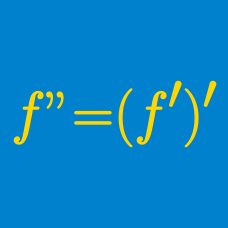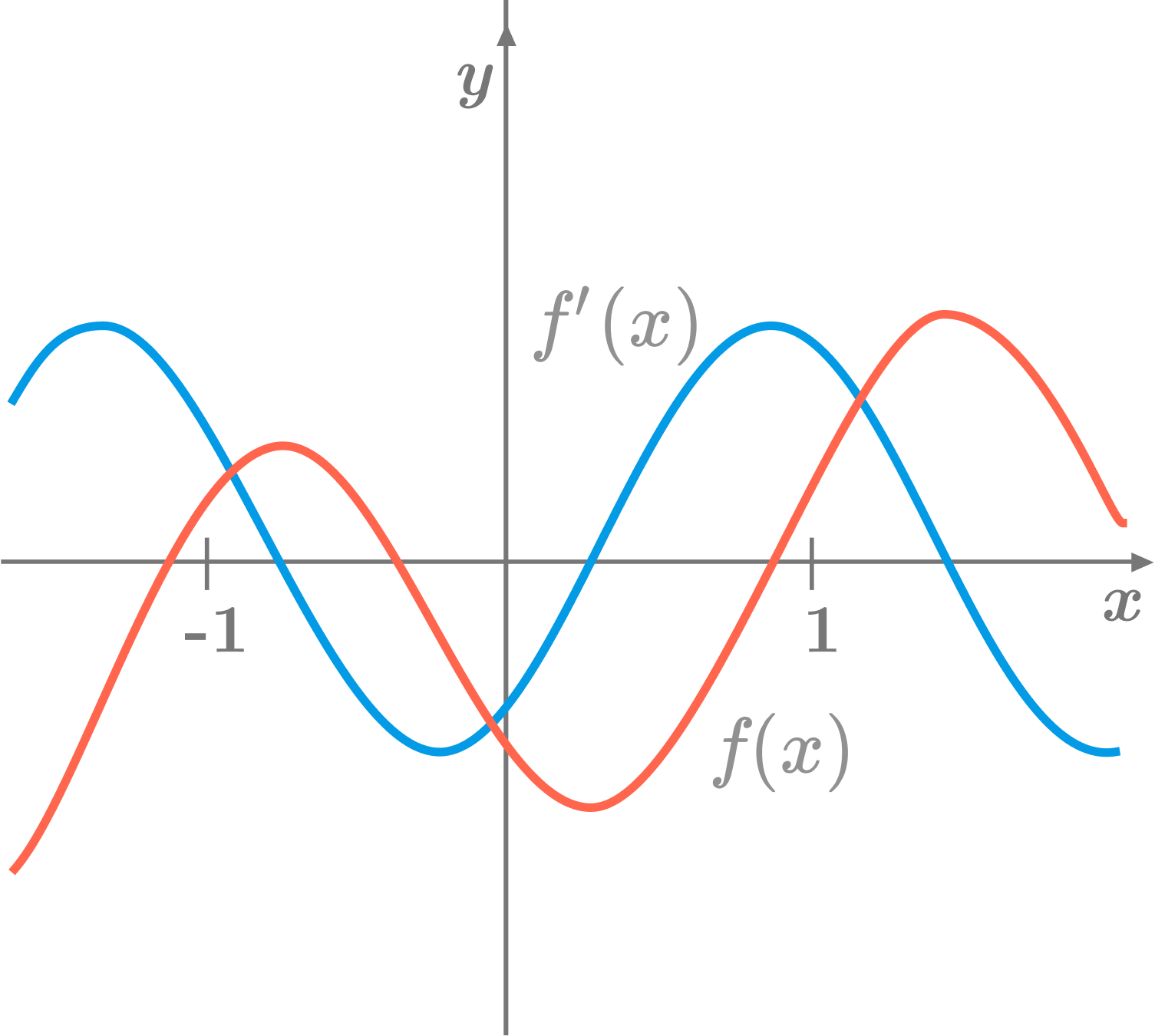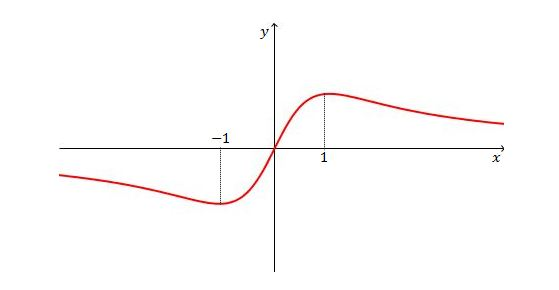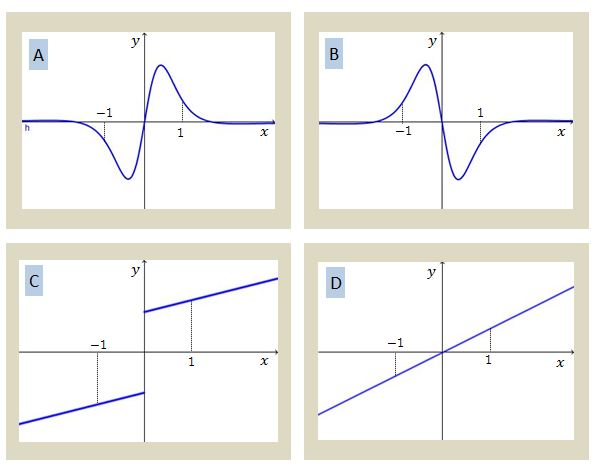Calculus

# Higher-order Derivatives: Level 1 Challenges

What is the fewest number of derivatives one needs to compute so that $$f(x) = 568{ x }^{ 499 }$$ becomes zero?In the above diagram, the red curve is the graph of a function $$f,$$ and the blue curve is the graph of its first derivative $$f'.$$ What is the relationship between $$f'(-1)$$ and $$f''(1) ?$$Given the graph of $$y=f(x)$$ above, which of the following is a possible graph of $$y=f''(x)?$$Let $$P$$ be a point moving in the $$xy$$-plane whose coordinates at time $$t$$ are given by $x(t)=2e^t\sin t, y(t)=6\cos t.$ What is the minimum value of the magnitude of the acceleration of $$P?$$

Find $$f^{(100)}(0)$$. If

$\large{ f(x) = \sin x + x^{100000}}$

Note: $$f^n(x)$$ denotes the $$n^{th}$$ derivative of $$f(x)$$.

×

Problem Loading...

Note Loading...

Set Loading...